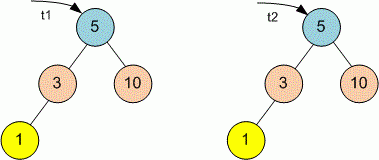favorite We need a little bit of your help to keep things running, click on this banner to learn more
Problems

# Tree Same

Given two binary trees, check if they are equal or not. Two binary trees are considered equal if they are structurally identical and the nodes have the same value.

Definition of a tree:

// Java
class TreeNode
{
public:
int val;
TreeNode left;
TreeNode right;
TreeNode(int x) {
val = x;
left = NULL;
right = NULL;
};

// C++
class TreeNode
{
public:
int val;
TreeNode *left;
TreeNode *right;
TreeNode(int x) : val(x), left(NULL), right(NULL) {}
};


Implement function isSame that returns true if trees are equal and false otherwise.

// Java
boolean isSame(TreeNode tree1, TreeNode tree2)

// C++
bool isSame(TreeNode *tree1, TreeNode *tree2)


#### ExampleFunction isSame returns true because the trees are equal.

Time limit 1 second
Memory limit 128 MiB
Author Mykhailo Medvediev